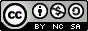Journal topic
Hydrol. Earth Syst. Sci., 7, 680–692, 2003
https://doi.org/10.5194/hess-7-680-2003Hydrol. Earth Syst. Sci., 7, 680–692, 2003
https://doi.org/10.5194/hess-7-680-200331 Oct 2003

31 Oct 2003

# Reduction of Monte-Carlo simulation runs for uncertainty estimation in hydrological modelling

S.-T. Khu1 and M. G. F. Werner2 S.-T. Khu and M. G. F. Werner
• 1University of Exeter, Harrison Building, North Park Road, Exeter, EX4 4QF, UK
• 2Delft University of Technology, P.O.Box 5048, 2600 GA, The Netherlands
• Email for corresponding author: s.t.khu@exeter.ac.uk

Abstract. Monte-Carlo (MC) simulation based techniques are often applied for the estimation of uncertainties in hydrological models due to uncertain parameters. One such technique is the Generalised Likelihood Uncertainty Estimation technique (GLUE). A major disadvantage of MC is the large number of runs required to establish a reliable estimate of model uncertainties. To reduce the number of runs required, a hybrid genetic algorithm and artificial neural network, known as GAANN, is applied. In this method, GA is used to identify the area of importance and ANN is used to obtain an initial estimate of the model performance by mapping the response surface. Parameter sets which give non-behavioural model runs are discarded before running the hydrological model, effectively reducing the number of actual model runs performed. The proposed method is applied to the case of a simple two-parameter model where the exact parameters are known as well as to a widely used catchment model where the parameters are to be estimated. The results of both applications indicated that the proposed method is more efficient and effective, thereby requiring fewer model simulations than GLUE. The proposed method increased the feasibility of applying uncertainty analysis to computationally intensive simulation models.

Keywords: parameters, calibration, GLUE, Monte-Carlo simulation, Genetic Algorithms, Artificial Neural Networks, hydrological modelling, Singapore